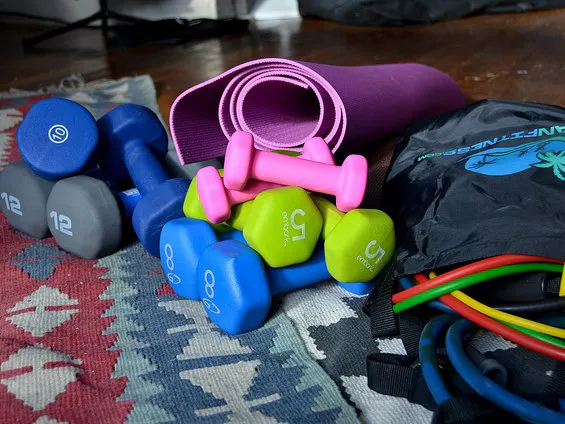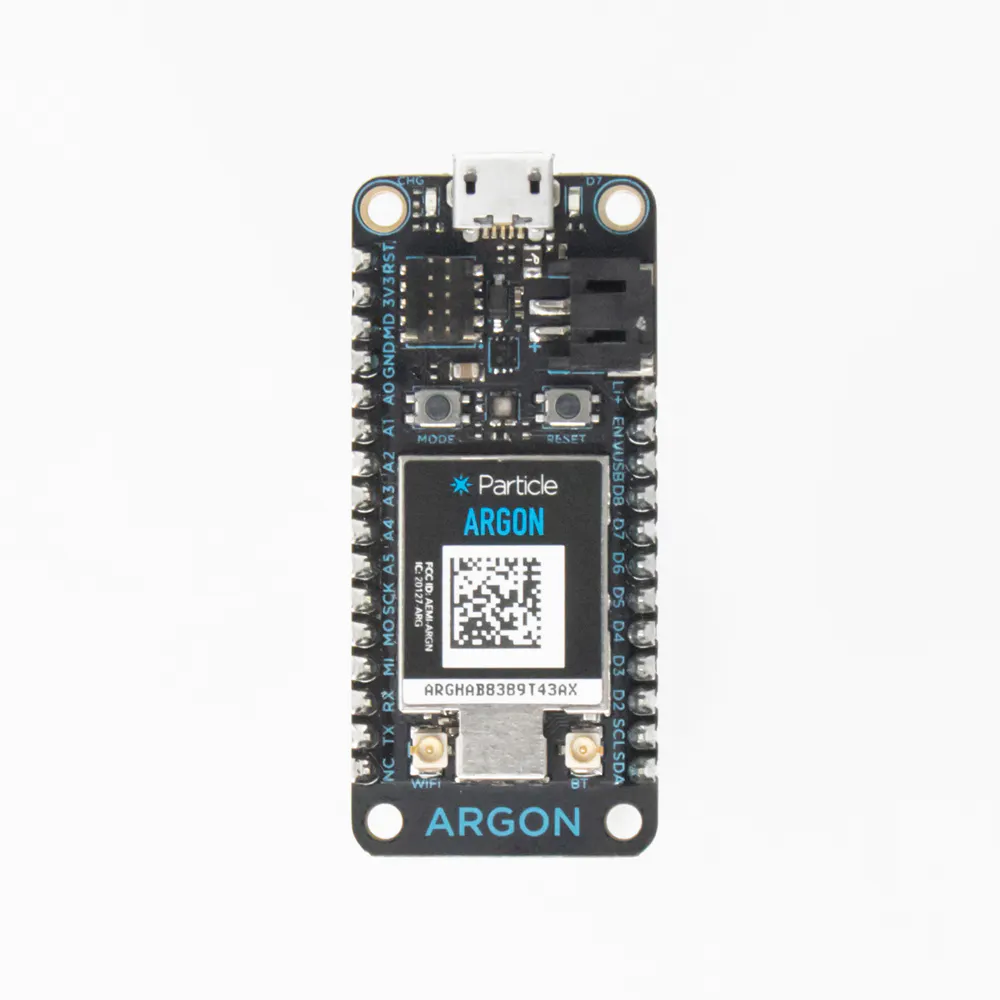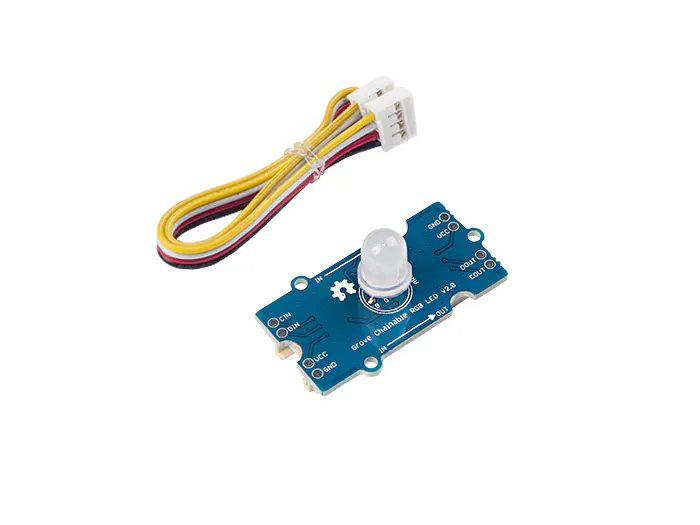Published

# IOT Fitness Tracker with Particle Argon

Track pushup, pullup, sit up, or squat reps and share with your workout partner - virtually!

BeginnerFull instructions provided1,398## Things used in this project

### Hardware componentsParticle Argon
×1Seeed Studio Grove – Chainable RGB Led V2.0
×1Ultrasonic Sensor - HC-SR04 (Generic)
×1

## Code

### Code of 1st Particle Argon

C/C++
```#include <Grove-Ultrasonic-Ranger.h>

float distance1 = 8.0;            // distance you must be from the senor to complete a full rep
int counter = 0;
int led_counter = 1;
int i;

Ultrasonic ultrasonic(D4);
void setup()
{
Serial.begin(9600);                         // Begin serial communications
pinMode(D2, OUTPUT);                        // Configure pin for output
pinMode(D3, OUTPUT);                       // Configure pin for output
pinMode(D7, OUTPUT);                       // Configure pin for output
pinMode(D7, OUTPUT);
digitalWrite(D2,HIGH);                    // this makes sure D2 has a starting value
Particle.subscribe("team7/wolfe/counter", pushupcounter);    //looking at the other particle to see if they did their reps

}

void pushupcounter(const char *event, const char *data){    //this is what runs when they complete there reps
{
i++;
Serial.print(i);
Serial.print(event);
Serial.print(", data: ");
if (data)
Serial.println(data);

else
Serial.println("NULL");

delay(500);
digitalWrite(D7, HIGH); //turns blue light on
}
}

void loop()
{

long RangeInCentimeters;                                                          // Variable to hold the distance measurement
RangeInCentimeters = ultrasonic.MeasureInCentimeters();                           // Retrieves the current distance value
Serial.print(RangeInCentimeters);                                                 // Out put the value over serial
Serial.println(" cm");                                                            // use 'particle serial monitor --follow' in the CLI to see serial output

if (RangeInCentimeters > distance1){                                              // If distance is greater than distance1
digitalWrite(D3, HIGH);                                                       // Red led on
digitalWrite(D2, LOW);                                                        // Green led off
led_counter = 0;

}

else if (RangeInCentimeters < distance1) {                                        // If distance is less than distance1
digitalWrite(D2, HIGH);                                                       // Green led on
digitalWrite(D3, LOW);                                                        // Red led off

if (led_counter == 0) {                                                       // the led_counter references the variable from the end of the previous loop and compares it to the output of D2 during the current loop. If they are the same (D2 = HIGH) then no rep will be counted. But if rep_counter = LOw, this means
counter = counter +1;
Particle.publish("repcomplete", String(counter));                          // publishing every rep you complete
delay(500);

}

else if (led_counter == 1){
counter = counter;
}

led_counter = 1;
}

if (counter == 5){                                                           // when the reps = 5 the particle will publish you did it
Particle.publish("team7/salo/counter", "youdiditsalo");
delay(500);
}

}
```

### Code of 2nd Particle Argon

C/C++
```#include <Grove-Ultrasonic-Ranger.h>

float distance1 = 8.0;            // distance you must be from the senor to complete a full rep
int counter = 0;
int led_counter = 1;
int i;

Ultrasonic ultrasonic(D4);
void setup()
{
Serial.begin(9600);                         // Begin serial communications
pinMode(D2, OUTPUT);                        // Configure pin for output
pinMode(D3, OUTPUT);                       // Configure pin for output
pinMode(D7, OUTPUT);                       // Configure pin for output
pinMode(D7, OUTPUT);
digitalWrite(D2,HIGH);                    // this makes sure D2 has a starting value
Particle.subscribe("team7/salo/counter", pushupcounter);    //looking at the other particle to see if they did their reps

}

void pushupcounter(const char *event, const char *data){    //this is what runs when they complete there reps
{
i++;
Serial.print(i);
Serial.print(event);
Serial.print(", data: ");
if (data)
Serial.println(data);

else
Serial.println("NULL");

delay(500);
digitalWrite(D7, HIGH); //turns blue light on
}
}

void loop()
{

long RangeInCentimeters;                                                          // Variable to hold the distance measurement
RangeInCentimeters = ultrasonic.MeasureInCentimeters();                           // Retrieves the current distance value
Serial.print(RangeInCentimeters);                                                 // Out put the value over serial
Serial.println(" cm");                                                            // use 'particle serial monitor --follow' in the CLI to see serial output

if (RangeInCentimeters > distance1){                                              // If distance is greater than distance1
digitalWrite(D3, HIGH);                                                       // Red led on
digitalWrite(D2, LOW);                                                        // Green led off
led_counter = 0;

}

else if (RangeInCentimeters < distance1) {                                        // If distance is less than distance1
digitalWrite(D2, HIGH);                                                       // Green led on
digitalWrite(D3, LOW);                                                        // Red led off

if (led_counter == 0) {                                                       // the led_counter references the variable from the end of the previous loop and compares it to the output of D2 during the current loop. If they are the same (D2 = HIGH) then no rep will be counted. But if rep_counter = LOw, this means
counter = counter +1;
Particle.publish("repcomplete", String(counter));                          // publishing every rep you complete
delay(500);

}

else if (led_counter == 1){
counter = counter;
}

led_counter = 1;
}

if (counter == 5){                                                           // when the reps = 5 the particle will publish you did it
Particle.publish("team7/wolfe/counter", "youdidit");
delay(500);
}

}
```

## Credits

### Caleb Salo

1 project • 1 follower

### Leland Wolfe II

1 project • 1 follower